## Sunday, November 10, 2013

### How to visualize test change scores for coaches

How to visualize test change scores for coaches

# How to visualize test change scores for coaches

## Introduction

Coaches are not interested in making inferences to a population, statistical significance, nor mean values (P<0.01). What they are interested are individual athletes, their reactions to training and what are practically significant and meaningful changes.

Before proceeding reading this how-to blog post, I urge readers to read the following two presentations from Will Hopkins

If you have read those must read presentations then we can continue. If you haven't please do.

To evaluate change (is it practically meaningful) we are going to use SWC (Smallest Worthwhile Change) and TE (Typical Error). If you don't know what they are and how they are used then you haven't read the mentioned Will Hopkin's presentations.

Knowing SWC and TE for a test and/or individual (in this case test) we can use NOVEL way of visualizing test change score for the reports so that coaches can understand and see individual players reaction and whether it practically meaningful

## Calcuating Typical Error for the test/estimate

I beleive in in-house research, at least doing something simple as this. Knowing typical error (TE) for the test/estimate is of great importance to judge the real change.

What does this mean in real world? It means that if we test a player in vertical jump one day when he was fully rested, and then we re-test him couple of days after in same conditionins (with the assumption that there are no learning effects nor improvement happening; that's why it is important to do familiarization with the test first) the scores might differ a little. This might be due measurement error and/or biological variability.

Then if we test that same player couple of months later to see the effects of training, how certain are we that the change is real? Here comes TE to help out as you will see later in the visualization and calculus of harmful/trivial/beneficial changes.

Let's write a function in R that calculates TE. But first we need to simulate some testing scores.

``````# Load library for random names generation for athlete
library(randomNames)

# Simulate vertical jump scores for 10 players, with an average of 55cm and
# SD of 6cm We are going to repeat the assessment on two days in the same
# conditionins and add some 'noise'
set.seed(505)
reliabilityTest1CM <- rnorm(10, mean = 55, sd = 6)
reliabilityTest2CM <- reliabilityTest1CM + runif(10, min = -2, max = 2)

# Let's name those 10 athletes
reliabilityPlayerNames <- randomNames(10)

# Put the data in data frame
reliabilityData <- data.frame(Athlete = reliabilityPlayerNames, Test1 = reliabilityTest1CM,
Test2 = reliabilityTest2CM)

# Calcualte the differences between tests
reliabilityData <- within(reliabilityData, Diff <- Test2 - Test1)
``````

Here are our simulated reliability test scores

Athlete Test1 Test2 Diff
1 Leyva-Fernandez, Kelsey 48.27 46.33 -1.95
2 Fernandez-Betance, Andrew 47.31 47.56 0.26
3 Cochran, Rayahna 42.76 42.38 -0.39
4 Darnell, Fabian 49.37 49.36 -0.01
5 Zahid, Angie 51.94 51.20 -0.74
6 Davids, Gina 52.52 52.37 -0.15
7 Trimble, Lalicia 49.54 49.22 -0.32
8 Harvie, Iaasic 55.84 57.26 1.41
9 Silas, Eduardo 58.39 59.13 0.74
10 Vargas, Mitchell 52.41 54.12 1.71

Now let's code the function that calculates the TE from data frame. I won't be using log transformations in this how-to, and to be honest I don't completely understand it (yet). In the near future definitely (hey, I am learning as well)

``````typicalError <- function(data, test1 = "Test1", test2 = "Test2", na.rm = TRUE) {
# Remove the missing values
if (na.rm)
data <- na.omit(data)

# Calculate the difference from named collums
diff <- data[[test2]] - data[[test1]]

return(sd(diff)/sqrt(2))
}
``````

In our simulated reliability study TE is 0.75cm. What the hell does that mean? To get some insight into it's magnitude we need to compare it to SWC (smallest worthwhile change). This way we compare signal vs. noise. SWC in our Test1 is 0.2 x SD or 0.89cm. If TE < SWC we have a decent test. If not we need to figure out the way to make it more reliable.

In our case:

• TE= 0.75cm
• SWC= 0.89cm
• TE < SWC Test is good

## Visualizing data for coaches

Now when we know SWC and TE for our tests we need to visualize those for the coaches to get clear and meaningful insight, and not only for team averages but for every single individual.

We are going to simulate pre- and post- data (e.g. beginning of pre-season and end of pre-season). For pre- data we are going to use already created data for our reliability study (Test1) and for post- data we are going to assume improvement of 5 cm on average (from 55cm to 60cm, with SD 6cm).

``````# Create vertical jump data
verticalJumpCM <- data.frame(Athlete = reliabilityPlayerNames, Pre = reliabilityTest1CM,
Post = rnorm(10, mean = 60, sd = 6))
``````

This is how our data set looks like

Athlete Pre Post
1 Leyva-Fernandez, Kelsey 48.27 54.11
2 Fernandez-Betance, Andrew 47.31 63.96
3 Cochran, Rayahna 42.76 56.56
4 Darnell, Fabian 49.37 55.01
5 Zahid, Angie 51.94 64.31
6 Davids, Gina 52.52 52.65
7 Trimble, Lalicia 49.54 57.49
8 Harvie, Iaasic 55.84 69.50
9 Silas, Eduardo 58.39 62.01
10 Vargas, Mitchell 52.41 50.57

Now we need to write a function that draws this data in a meaningful way. I am going to use Box Plots and put the names of each player on top of them. Besides that we are going to color-code each athlete depending if he had harmful, trivial or beneficial change. The function would need to calculate SWC and use that as a zone. If a given player did not cross SWC, then he show trivial improvement. If he improved over or under SWC he showed beneficial or detrimentral change respectively.

The beneficial or harmful zones depends on type of estimate - higher score is better or lower score is better. To take this into account I will put special flag in the function so it knows the difference.

``````# Load reshape2 library for melt fucntion (from wide to long format
# convertor) and ggplot2 for plotting
library(reshape2)
library(ggplot2)

coachingBoxPlot <- function(data, athlete = "Athlete", pre = "Pre", post = "Post",
meanFrom = pre, SWCFrom = pre, SWCFactor = 0.2, inverse = FALSE, beneficial = "Beneficial",
trivial = "Trivial", harmful = "Harmful", na.rm = TRUE, title = "Pre and Post",
valueName = "", beneficialColor = "black", trivialColor = "orange", harmfulColor = "red",
boxColor = "grey", drawSWC = FALSE, SWCColor = "yellow") {

# Remove the missing values
if (na.rm)
data <- na.omit(data)

# Calculate differences from post and pre
diff <- data[[post]] - data[[pre]]

# Calculate mean from data
dataMean = mean(data[[meanFrom]])

# Calculate SWC from data
SWC <- sd(data[[SWCFrom]]) * SWCFactor
SWCLower <- dataMean - SWC
SWCUpper <- dataMean + SWC

# Calculate beneficial, trivial and harmful based on diff
if (inverse) {
data\$change <- trivial
data\$change[diff > SWC] <- harmful
data\$change[diff < (-SWC)] <- beneficial
} else {
data\$change <- trivial
data\$change[diff > SWC] <- beneficial
data\$change[diff < (-SWC)] <- harmful

}

# melt the data from wide formt to long format that is used by ggplot
meltedData <- melt(data, id = c(athlete, "change"))

# Change the name of athlete collumn to 'athlete' for easier use by ggplot2
names(meltedData) <- "athlete"

# Reorder the factor levels in change score
if (inverse) {
meltedData\$change = factor(meltedData\$change, levels = c(harmful, trivial,
beneficial), ordered = TRUE)
} else {
meltedData\$change = factor(meltedData\$change, levels = c(beneficial,
trivial, harmful), ordered = TRUE)
}

# Create a boxplot using ggplot2
boxPlot <- ggplot(meltedData, aes(y = value, x = 1))
boxPlot <- boxPlot + geom_boxplot(fill = boxColor, alpha = 0.6)
boxPlot <- boxPlot + stat_summary(fun.y = mean, geom = "point", fill = "white",
shape = 23, size = 5)

boxPlot <- boxPlot + labs(x = NULL, y = valueName, fill = NULL, title = title)
boxPlot <- boxPlot + geom_text(aes(label = athlete, color = change), alpha = 1,
size = 3.5, position = position_jitter(height = 0, width = 0.3))

if (inverse) {
boxPlot <- boxPlot + scale_colour_manual(values = c(harmfulColor, trivialColor,
beneficialColor))
} else {
boxPlot <- boxPlot + scale_colour_manual(values = c(beneficialColor,
trivialColor, harmfulColor))
}

boxPlot <- boxPlot + facet_grid(. ~ variable) + scale_x_continuous(breaks = NULL)
boxPlot <- boxPlot + theme(legend.position = c(0.08, 0.9), legend.title = element_blank())

if (drawSWC) {
boxPlot <- boxPlot + geom_hline(yintercept = dataMean, linetype = 3)
boxPlot <- boxPlot + geom_rect(xmin = 0, xmax = 2, ymin = SWCLower,
ymax = SWCUpper, fill = SWCColor, alpha = 0.05)
}

return(boxPlot)

}
``````

Let's see out function in action

``````coachingBoxPlot(data = verticalJumpCM, valueName = "Vertical Jump [cm]", title = "Change in vertical jump height")
``````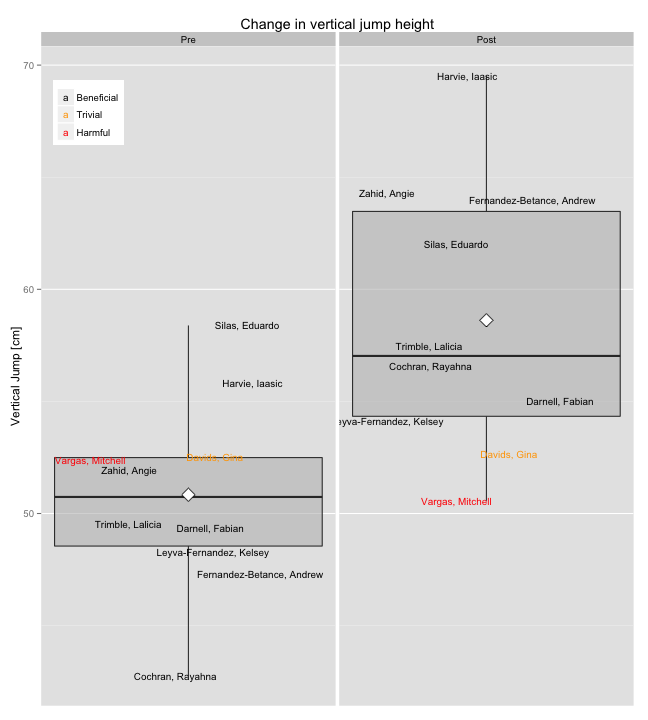We can also draw SWC for the group means, while also changing it to 0.5 x SD. Let's see what happens

``````coachingBoxPlot(data = verticalJumpCM, valueName = "Vertical Jump [cm]", title = "Change in vertical jump height",
drawSWC = TRUE, SWCFactor = 0.5)
``````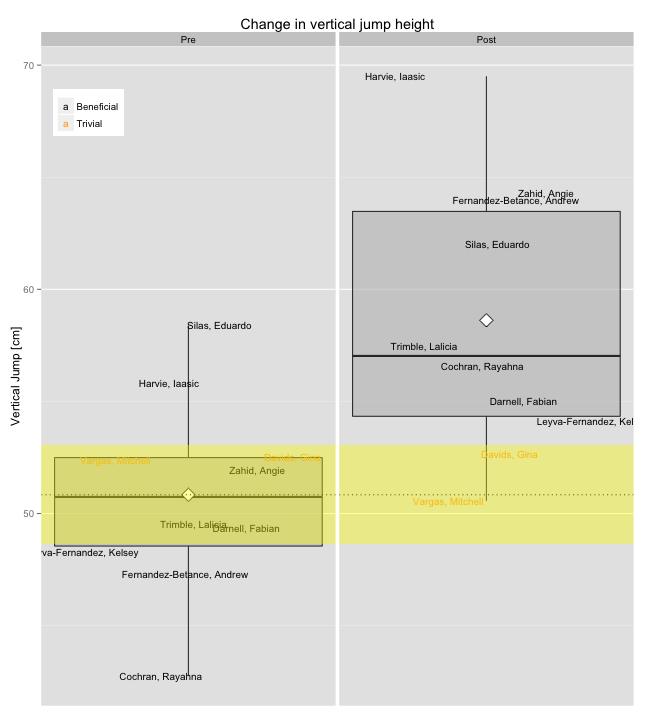Notice that we have more athletes with trivial change (by default it is colored in yellow)

With the above graph, coach can see full range of changes and can see visually the change of each athlete and wether it is beneficial, trivial or detrimental based. Suppose the coach wants to see more individual data regarding the magnitude of change?

Let's write another visualization.

``````
changePlot <- function(data, athlete = "Athlete", pre = "Pre", post = "Post",
TypicalError = 0, SWCFrom = pre, SWCFactor = 0.2, inverse = FALSE, beneficialColor = "green",
trivialColor = "yellow", harmfulColor = "red", na.rm = TRUE, title = "Test change",
valueName = "", dotColor = "blue", drawSWC = TRUE, SWCColor = trivialColor,
drawTE = TRUE, chances = TRUE, conf.level = 0.9, drawBands = FALSE) {

# Remove the missing values
if (na.rm)
data <- na.omit(data)

# Calculate differences from post and pre
diff <- data[[post]] - data[[pre]]

# Calculate SWC from data
SWC <- sd(data[[SWCFrom]]) * SWCFactor

# Create new data frame
changeData <- data.frame(athlete = data[[athlete]], diff = diff, TE = TypicalError)

# Sort the data
orderedElements <- order(changeData\$diff, decreasing = inverse)

changeData\$athlete <- factor(changeData\$athlete, levels = unique(changeData\$athlete[orderedElements]),
ordered = TRUE)
changeData <- changeData[orderedElements, ]

# Calculate the Z number
conf.level <- conf.level + (1 - conf.level)/2
Z <- qnorm(conf.level)

# Calculate confidence intervals
changeData\$LowConf <- changeData\$diff - (changeData\$TE * Z)
changeData\$HiConf <- changeData\$diff + (changeData\$TE * Z)

# Calculate Chances (See Will Hopkins)
changeData <- within(changeData, harmful <- pnorm(-SWC, mean = diff, sd = TE))
changeData <- within(changeData, trivial <- pnorm(SWC, mean = diff, sd = TE) -
harmful)
changeData <- within(changeData, beneficial <- 1 - (harmful + trivial))
changeData <- within(changeData, chances <- paste("[", as.character(round(harmful *
100, 0)), ",", as.character(round(trivial * 100, 0)), ",", as.character(round(beneficial *
100, 0)), "]", sep = ""))

# Count the number of athletes
athletesNumber = length(changeData\$athlete)

# Get maximums on x axis [used for drawing bands]
minX <- min(changeData\$LowConf)
maxX <- max(changeData\$HiConf)
rangeX <- maxX - minX
maxX <- maxX + rangeX * 0.05
minX <- minX - rangeX * 0.05

# Plot the data
gg <- ggplot(changeData, aes(x = diff, y = athlete))
gg <- gg + geom_point(size = 3, color = dotColor)
if (drawTE)
gg <- gg + geom_errorbarh(aes(xmin = LowConf, xmax = HiConf), height = 0.2)
gg <- gg + labs(y = NULL, x = valueName, fill = NULL, title = title)
gg <- gg + geom_vline(xintercept = 0, linetype = 3)
if (drawSWC)
gg <- gg + geom_rect(xmin = -SWC, xmax = SWC, ymin = 0, ymax = athletesNumber +
1, fill = SWCColor, alpha = 0.05)
if (chances)
gg <- gg + geom_text(aes(label = chances), alpha = 1, size = 2.5, vjust = -1.5,
hjust = 0)

if (drawBands) {
if (!drawSWC)
gg <- gg + geom_rect(xmin = -SWC, xmax = SWC, ymin = 0, ymax = athletesNumber +
1, fill = trivialColor, alpha = 0.05)

if (inverse) {
gg <- gg + geom_rect(xmin = minX, xmax = -SWC, ymin = 0, ymax = athletesNumber +
1, fill = beneficialColor, alpha = 0.05)
gg <- gg + geom_rect(xmin = SWC, xmax = maxX, ymin = 0, ymax = athletesNumber +
1, fill = harmfulColor, alpha = 0.05)
} else {
gg <- gg + geom_rect(xmin = minX, xmax = -SWC, ymin = 0, ymax = athletesNumber +
1, fill = harmfulColor, alpha = 0.05)
gg <- gg + geom_rect(xmin = SWC, xmax = maxX, ymin = 0, ymax = athletesNumber +
1, fill = beneficialColor, alpha = 0.05)
}
}
return(gg)
}
``````

Let see how this function works for our vertical jump data

``````# Get the TE from reliability 'study'
TE <- typicalError(reliabilityData)

changePlot(data = verticalJumpCM, TypicalError = TE, valueName = "Vertical Jump change [cm]")
``````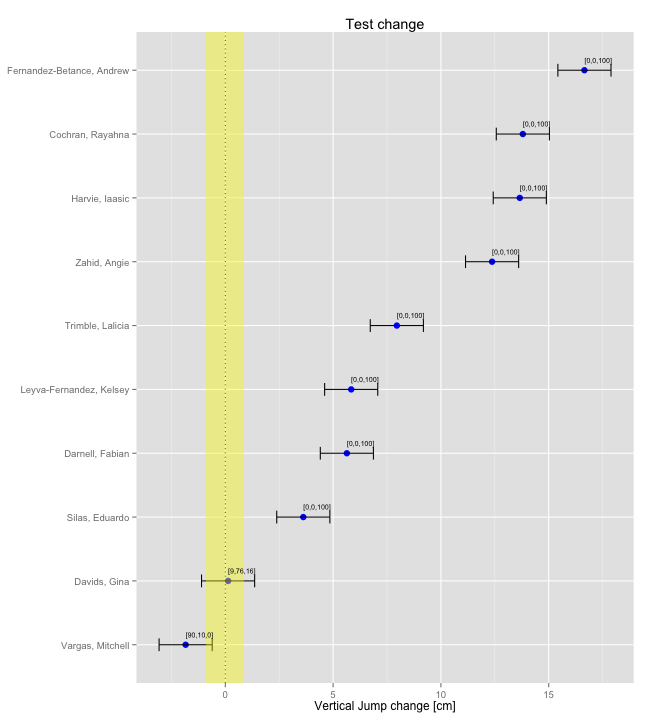What we got on the graph are sorted change scores with error bars that represent confidence intervals with 90% confidence level. Using function parameter we can change confidence interval (default is 90%). In the square brackets are calculated chances for Harmful, Trivial and Beneficial change. This concept is VERY important and needs to be visualized. Sometimes when TE is large, we are not sure if the change is real or just normal variability in performance.

We can simulate different TE for athletes to see the effect on chances, and put bands color (Harmful, Trivial, Beneficial) and also increase SWC to 0.5 x SD

``````changePlot(data = verticalJumpCM, TypicalError = 1:5, valueName = "Vertical Jump change [cm]",
drawBands = TRUE, SWCFactor = 0.5)
``````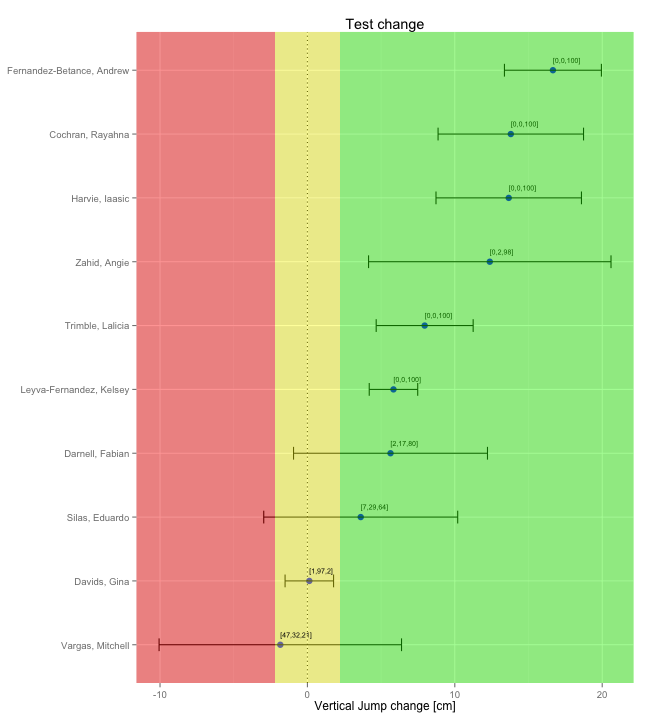To get the full picture we need to combine the box plot and change plot. This could be used to report changes in test.

``````library(gridExtra)
``````
``````## Loading required package: grid
``````
``````
plot1 <- coachingBoxPlot(data = verticalJumpCM, valueName = "Vertical Jump [cm]",
title = "Change in vertical jump height")

plot2 <- changePlot(data = verticalJumpCM, TypicalError = TE, valueName = "Vertical Jump change [cm]",
title = "Change in vertical jump height", chances = FALSE)

grid.arrange(plot1, plot2, ncol = 2)
``````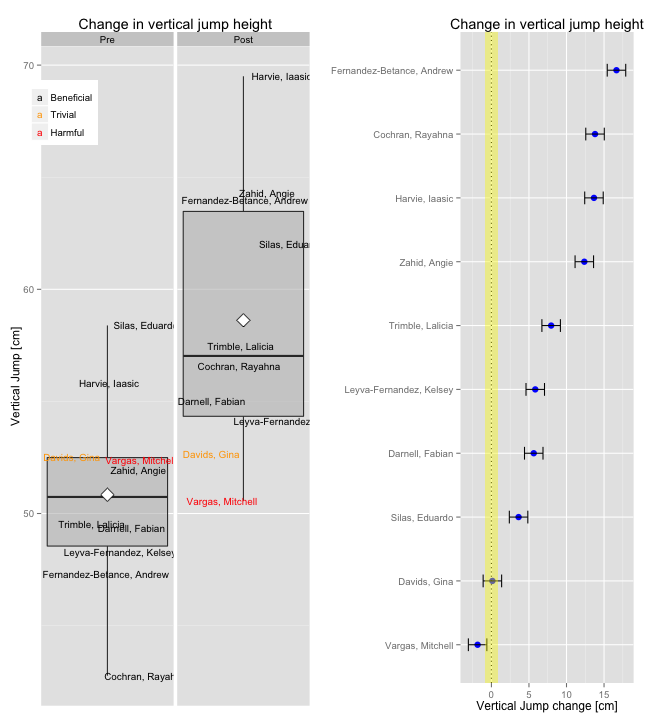This way coaches can see position of the player in squat both pre and post, along with his individual change. In my opinion this is the whole picture - you want to see the rank of a player and you want to see how much did he improved.

Another graph we can plot is Quadrant Plot, which shows the relative position (Z-score) in test score on x axis and relative position (Z-score) in change in test score. This way we can identify talents or those who have high rank in test score and who adapted the most.

Let's write the function

``````quadrantPlot <- function(data, athlete = "Athlete", pre = "Pre", post = "Post",
use = pre, na.rm = TRUE, title = "Quadrant Plot", xLabel = "Test [Z-score]",
yLabel = "Change[Z-score]", dotColor = "blue", quadrant1Color = "blue",

# Remove the missing values
if (na.rm)
data <- na.omit(data)

# Calculate differences from post and pre
diff <- data[[post]] - data[[pre]]

# Calculate difference Z score
diffZ <- (diff - mean(diff))/sd(diff)

# Calculate test Z score
testZ <- (data[[use]] - mean(data[[use]]))/sd(data[[use]])

# Create data frame
normalizedData <- data.frame(athlete = data[[athlete]], Test = testZ, Change = diffZ)

# Calculate x and y min and max
xMin <- min(normalizedData\$Test)
xMax <- max(normalizedData\$Test)
yMin <- min(normalizedData\$Change)
yMax <- max(normalizedData\$Change)
xRange <- xMax - xMin
yRange <- yMax - yMin

xMax <- xMax + xRange * 0.05
yMax <- yMax + yRange * 0.05
xMin <- xMin - xRange * 0.05
yMin <- yMin - yRange * 0.05

# Plot the data
gg <- ggplot(normalizedData, aes(x = Test, y = Change))
gg <- gg + geom_point(color = dotColor, size = 4)
gg <- gg + geom_vline(xintercept = 0, linetype = 1, size = 4, alpha = 0.5)
gg <- gg + geom_hline(yintercept = 0, linetype = 1, size = 4, alpha = 0.5)
gg <- gg + geom_text(aes(label = athlete), alpha = 1, size = 2.5, vjust = -1.5,
hjust = 0)
gg <- gg + labs(x = xLabel, y = yLabel, fill = NULL, title = title)

gg <- gg + geom_rect(xmin = xMin, xmax = 0, ymin = 0, ymax = yMax, fill = quadrant1Color,
alpha = 0.03)
gg <- gg + geom_rect(xmin = 0, xmax = xMax, ymin = 0, ymax = yMax, fill = quadrant2Color,
alpha = 0.03)
gg <- gg + geom_rect(xmin = xMin, xmax = 0, ymin = yMin, ymax = 0, fill = quadrant3Color,
alpha = 0.03)
gg <- gg + geom_rect(xmin = 0, xmax = xMax, ymin = yMin, ymax = 0, fill = quadrant4Color,
alpha = 0.03)
}

return(gg)
}
``````

This is how Quadrant plot looks like

``````quadrantPlot(data = verticalJumpCM, xLab = "Vertical Jump score Pre-test [Z score]",
yLab = "Vertical Jump Improvement")
``````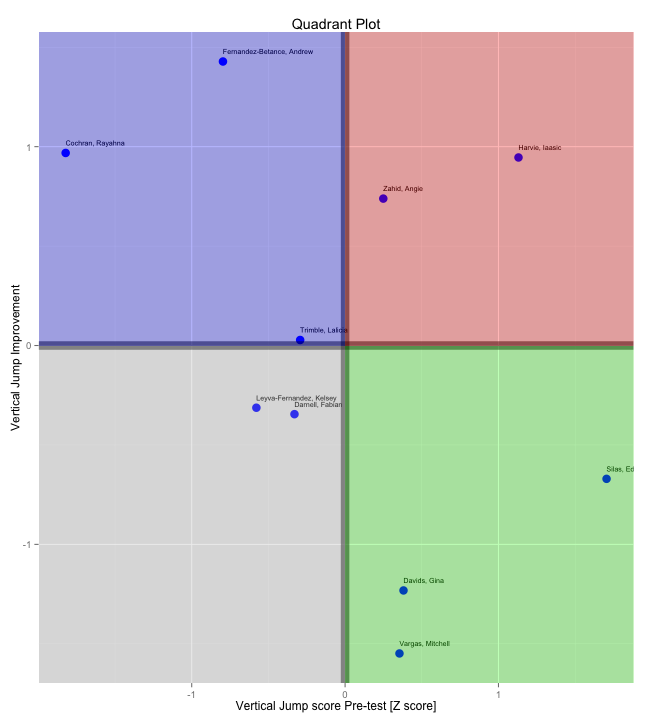With quadrant plot we can identify “outliers” and potential talents/hardgainer. In the second quadrant (red) we have athletes who scored more than average and improved more than average. In the third quadrant (grey) we have athletes that score less than average and improved less than average. These two groups are on the opposite end and migth need re-evaluation of the training program. Blue quadrant are the athletes who scored less than average, but improved more than average. In green quadrant we have athletes that scored more than average, but improved less than average.

The test score in this case is PRE test.

Using all mentioned three graphs we acquire lot more pragmatic value, because we can see who need different approach than used (individualization).

``````plot1 <- coachingBoxPlot(data = verticalJumpCM, valueName = "Vertical Jump [cm]",
title = "Change in vertical jump height")

plot2 <- changePlot(data = verticalJumpCM, TypicalError = TE, valueName = "Vertical Jump change [cm]",
title = "Change in vertical jump height", chances = FALSE)

plot3 <- quadrantPlot(data = verticalJumpCM, xLab = "Vertical Jump score Pre-test [Z score]",
yLab = "Vertical Jump Improvement")

grid.arrange(plot1, plot2, plot3, nrow = 3)
``````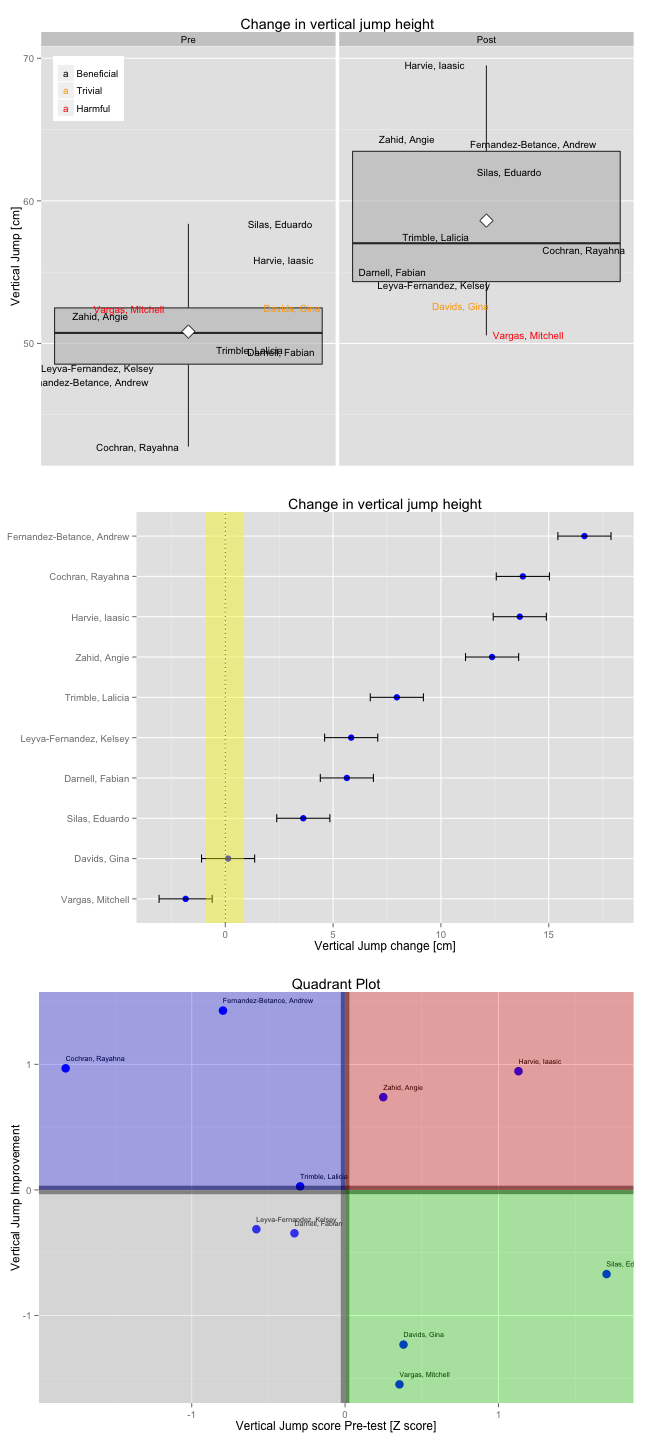Ok, now we have visualized changes in two test. One more visualization technique we would need is to visualize time-series, or changes in test scores over time for each athlete. This one should involve SWC plotted around starting point and plotted TE (Confidence Intervals) for every observation.

It is simple to do it for a single athlete, but I want to plot all of them at the same time. This involves some tricks with ggplot2 which I still don't know :(

This is what I was able to do for now.

Generate some time-series data for players

``````# Take the name of our athletes from simulated data
athleteNames <- unique(verticalJumpCM\$Athlete)
potentialDates <- seq(as.Date("2013/1/1"), as.Date("2014/1/1"), by = "1 day")

# Generate new data
sampledAthletes <- sample(athleteNames, size = 500, replace = TRUE)
sampledDates <- sample(potentialDates, size = 500, replace = TRUE)
sampleScores <- runif(500, min = 40, max = 60)
verticalJumpCM <- data.frame(Athlete = sampledAthletes, Date = sampledDates,
verticalJump = sampleScores)
``````

Now let's write a function that visualize it

``````# for this function data needs to be in long format as opposed to two others
longitudinalDataChart <- function(data, title = "") {
gg <- ggplot(data, aes(x = Date, y = verticalJump, color = Athlete))
gg <- gg + geom_line()
gg <- gg + geom_point(size = 1.5)
gg <- gg + facet_grid(Athlete ~ .)
return(gg)
}
``````

And finally visualize it

``````longitudinalDataChart(verticalJumpCM)
``````